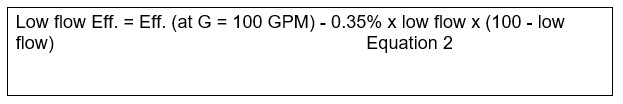# How to Estimate Pump Efficiency

In this post I want to share to you how to estimate pump efficiency. An equation was developed by Carl Branan, author of the Process Engineer’s Pocket Handbook. Pump efficiency can be estimated by using equation below:Where:

Eff.         = Pump percentage efficiency

G             = Flow (GPM)

Range of applicability:

F              = 50-300 ft

G             = 100-1000 GPM

Equation 1 above gives results within about 7% of the aforementioned pump curves. This mean within 7% of the curve value. If the curve value is 50%, the equation will be within the range 50 ± 3.5%.

For flow in the range of 25-99 GPM, a rough estimation can be obtained by using Equation 1 for 100 GPM and then subtracting 0.35% per GPM times the difference between 100 GPM and the low flow GPM. For flows at the bottom of the range (25-30 GPM), this will give results within about 15% for the middle of the head range and 25% at the extremes. This is adequate for ballpark estimates at these low flows. The Equation is below:Range of applicability:

F              = 50-300 ft

G             = 25-99 GPM

To make it easier, I made two charts to represent Equation 1 and Equation 2, respectively. Pump efficiency for developed head (F) between 50 and 300 ft and flow (G) between 100 and 1000 GPM is shown in Chart 1 below.

Chart 2 shows pump efficiency for developed head (F) between 50 and 300 ft and flow (G) between 25-90 GPM.

1.pik says: